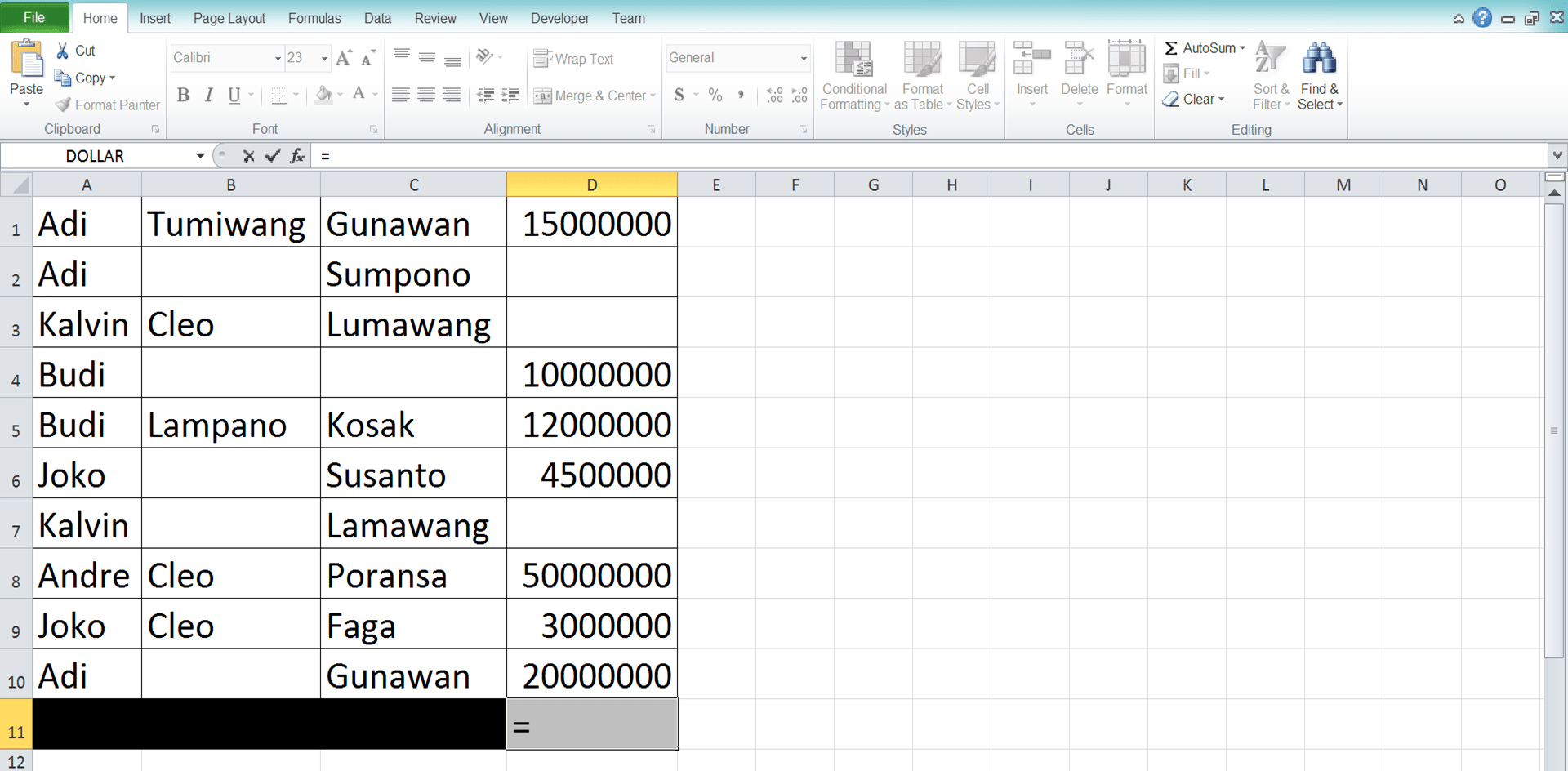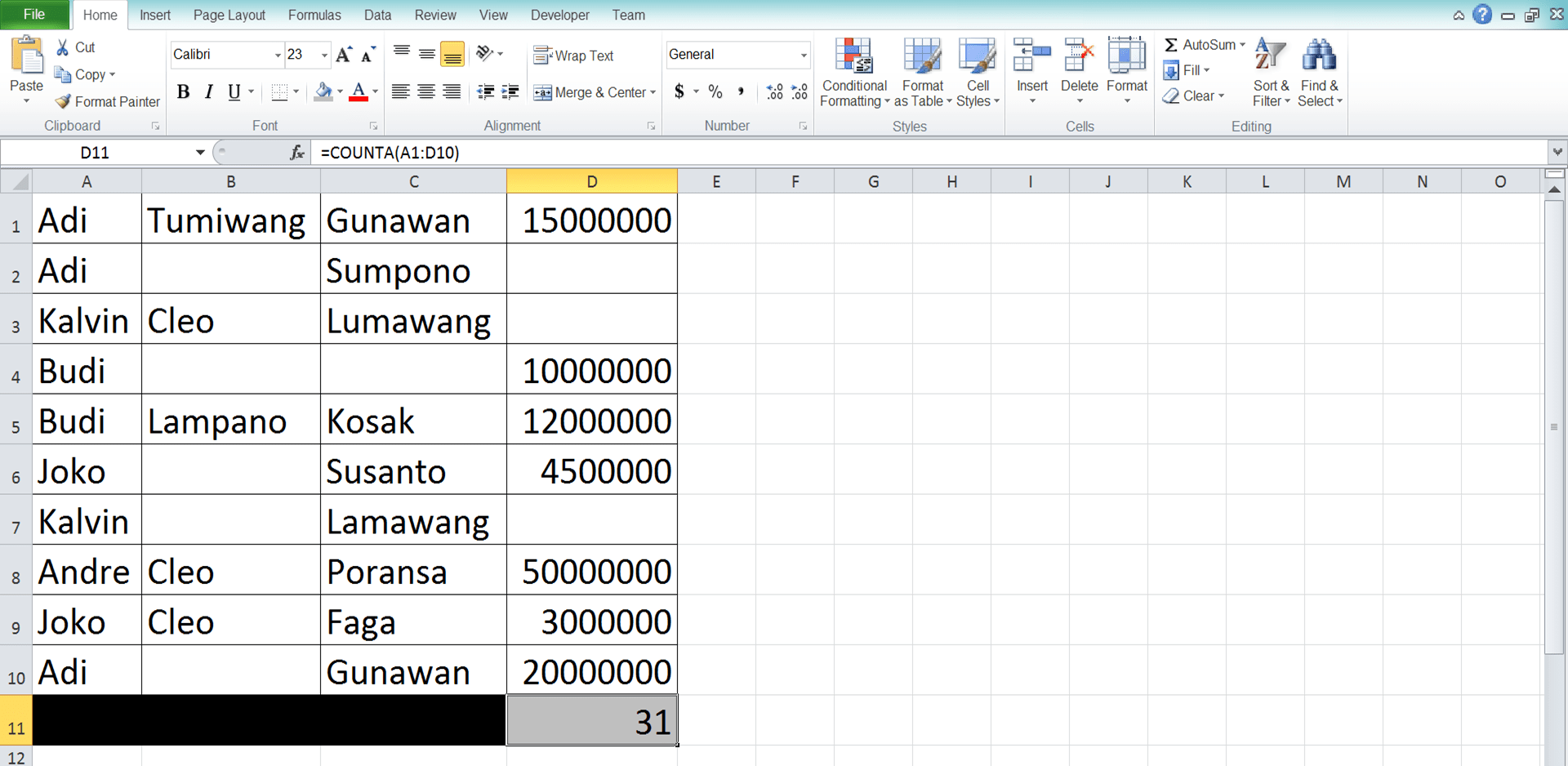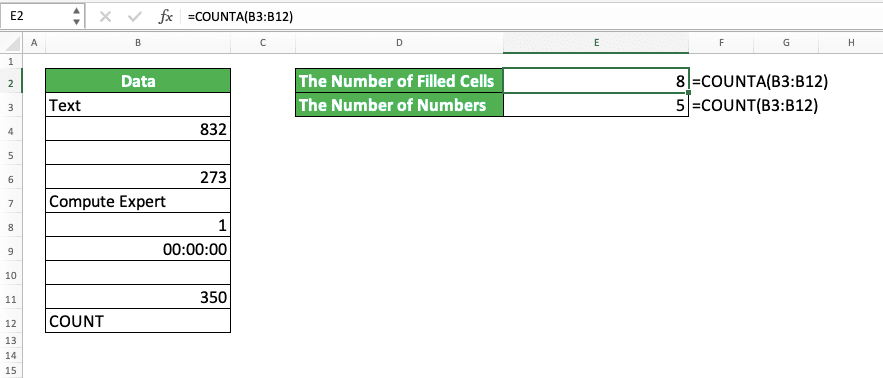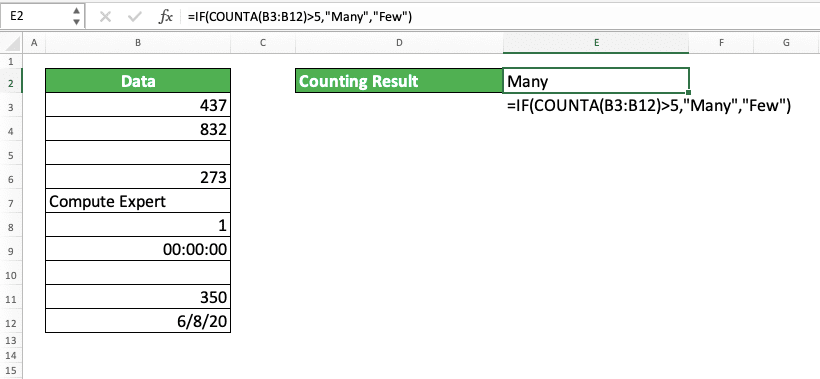COUNTA Excel Formula: Functions, Examples, and How to Use - Compute Expert

# COUNTA Excel Formula: Functions, Examples, and How to Use

Home >> Excel Tutorials from Compute Expert >> Excel Formulas List >> COUNTA Excel Formula: Functions, Examples, and How to Use

In this tutorial, you will learn the usage of the COUNTA excel formula completely, from its basic to its advanced use.

Counting the amount of data we have is one of the things we often need to do in excel. COUNTA can help you to do that fast if you know how to use the formula.

Curious to understand more about COUNTA? Read all parts of this tutorial to master its usage in excel!

Disclaimer: This post may contain affiliate links from which we earn commission from qualifying purchases/actions at no additional cost for you. Learn more

## What is COUNTA?

The definition of COUNTA is a formula you can use to count the amount of data you have.

In practice, we almost always use COUNTA to count the number of non-empty cells (or, in other words, the cells that contain data) in an excel cell range.

## COUNTA Function in Excel

We can use COUNTA to help us count how much data that we have in the inputs we give to it. We can give inputs to COUNTA in the form of direct data typing, cell coordinates, or cell ranges (that said, we almost always only give one cell range as our COUNTA input).

## COUNTA Result

COUNTA result is a number that represents the amount of data that we have from the inputs we give to it.

## Excel Version from Which We Can Start Using COUNTA Excel Formula

We can start using COUNTA since excel 2003.

## The Way to Write It and Its Inputs

Generally, the COUNTA writing form is like this in excel.

= COUNTA ( value1 , [ value2 ] , … )

The input of value1, [value2], … there is from where you want to count the amount of data from. You can input direct data, cells, or cell ranges there although we almost always input a cell range.

If you give more than one input to your COUNTA, then you must separate them with comma signs ( , ).

## Example of Its Usage and Result

You can see a COUNTA implementation and result example in the following.In the example, we can see how the COUNTA formula is utilized. You just need to input the data/cell coordinates/cell ranges you want to know the amount of data from. If you give more than one input, then make sure you use comma signs ( , ) in between your inputs.

This formula will return the amount of data counted in your inputs and ignore empty cells. COUNTA will count all kinds of data you have as you can see in the example. Either it is a number, text, date, or other types of data.

It is important to note that COUNTA will only ignore really empty cells in its counting process. The cells with formulas that produce empty data (usually represented with double quotes ( “” ) ) or cells that only contain spaces are also counted by COUNTA (be careful with these kinds of cells, though, as you will see why in some point next in this tutorial).

## Writing Steps

After discussing the COUNTA example, the following will explain how to write the formula in excel.

If you often use other formula variants to count data like COUNT or COUNTBLANK, then this should be easy. This is because the writing of those formulas and COUNTA is almost similar.

1. Type an equal sign ( = ) in the cell where you want to put your COUNTA result2. Type COUNTA (can be with large and small letters) and an open bracket sign after =3. Input the data/cell coordinates/cell ranges that you want to calculate the amount of data from. Input comma signs between all of your inputs4. Type a close bracket sign after you have given all your inputs to COUNTA5. Press Enter
6. Done!## Difference Between COUNT and COUNTA

This is something that a number of excel users can often be wrong about. When they want to count the data they have in a cell range, they use COUNT instead of COUNTA.

As the formula name (COUNT) indicates that it is something you can use to count data, this often causes its wrong usage. As a result, the ones who use COUNT to count their data get their results wrong!

When you need to count data in your cell range, you should use COUNTA instead. To make it clear, here is the difference between COUNT and COUNTA. You use COUNT to count numbers only while you use COUNTA to count all types of data!

To illustrate their differences clearer, here is an illustration of both implementations to the same excel cell range.You can see the difference between the COUNT and COUNTA results in the screenshot above. That is because COUNT and COUNTA count two different things!

COUNT only counts numbers while COUNTA counts all types of data. As a result, in the example above, COUNT produces 5 (as there are 5 numbers in the data column cell range) while COUNTA produces 8 (as there are 8 filled cells with data in the data column cell range)!

If you use COUNT, then note that date and time data is also counted as a number in excel. In the screenshot, you can see that COUNT also counts the time data in its cell range.

## COUNTA Users Beware: Cells that Look Empty But Actually Are Not

Using COUNTA but gets confused with the result that you get?

COUNTA may sometimes seem to count more data than it should be to you. When you try to check, there seems to be less data than what COUNTA tells you in your cell range.

If that is the case, then you need to be careful. You may have some cells that look empty but actually aren’t!

As mentioned briefly in the previous explanation, there are cells that may contain spaces or formulas that produce empty data ( “” ). They might look empty to us but because they actually have something in them, COUNTA counts them!

As an illustration of this, take a look at the screenshot below.You can see that the filled cells in the data column there seem to be only five. However, COUNTA gives us 8 instead when we try to count the data.

Why does that happen? It is because there are two cells there that contain only spaces and one cell which contains an empty result formula! That causes COUNTA to count them too and produces 8 instead of 5 expected.

If you want to remove those “problem” cells from your COUNTA counting process, then you need to delete their contents.

## Method to Produce an Outcome Based on a COUNTA Result: IF COUNTA Excel Formula

One of the most combined formulas with another formula in excel is IF. When you need to produce an outcome based on your COUNTA result, you need to combine your COUNTA with IF too.

For this, we make our COUNTA as part of the logic condition input of our IF. The general writing form of these two formulas combination is as follows.

= IF ( COUNTA ( value1, [ value2 ] , … ) condition , result_if_true , result_if_false )

As COUNTA produces a number, the condition you type after you write your COUNTA needs to be number-related too. Usually, it is a less than, more than, and/or equal to condition.

Your COUNTA will count the data from your inputs and your condition will test its counting result. If it fits, then you will get your IF TRUE result. If not, then you will get your IF FALSE result. Because of this process, you will get an outcome depending on your COUNTA result as intended!

To better understand this IF and COUNTA combination, here is its implementation example in excel.In this example, we try to mark the result of our COUNTA result, whether it is few or many. “Few” is if the result is <=5 while “Many” is if the result is >5.

To get that mark we want easier, we use the IF and COUNTA combination.

The formula writing is as you can see in the screenshot. We input COUNTA to our IF to make its result a part of our IF logic condition input. Next, we type the condition of “>5” there.

If the COUNTA result fulfills the condition (more than 5), then it will produce “Many” text. If not, then “Few” text is the outcome.

For the result of the example, we get a “Many” mark because our COUNTA result is 8 there!

## COUNTA to Count Data that Fulfill Specific Criteria: COUNTIFS

Need to count data in your cell range that fulfills specific criteria? Instead of trying to use COUNTA for that, you should use COUNTIFS.

COUNTIFS is a formula that excel specifically provides to count data that fulfill our specific criteria. The general writing form of COUNTIFS is like this.

= COUNTIFS ( criteria_range1 , criteria1 , … )

The inputs you give to COUNTIFS are considered in pairs. Each of the pairs contains a cell range (usually a column/row) and the criterion that will evaluate data in the cell range.

You need to input the number of those pairs in COUNTIFS according to the number of criteria you have. Excel will count the data entries that fulfill all the criteria you have in all their cell ranges.

Because of this counting process, it will be better if you input parallel and same-sized cell ranges to COUNTIFS. We usually input cell ranges in the form of columns or rows in COUNTIFS.

To better understand the concept of COUNTIFS, you can see its implementation example in excel below.In this example, we have three criteria for the data entries we want to count. We want to count the entries with B as the first answer, A as the second, and C as the third. We can use COUNTIFS to get this counting result much faster and easier for us.

Just input the column cell ranges of answer 1, 2, and 3 with their corresponding criteria. Input them in correct order in the COUNTIFS with the cell range first followed by its criterion.

Do that and we can get the number of our data entries that fulfill our criteria! As you can see in the example, we get 3 there as the COUNTIFS result (which means 3 respondents give B for the first answer, A for the second, and C for the third).

## COUNTA for a Data Table that Has Been Filtered: SUBTOTAL

What if we want to count data that passes the filter we apply in our data table? Can we do that using COUNTA?

Unfortunately, if you use COUNTA, then it will count the data which the filter hides too. For this counting process, you need to use the SUBTOTAL formula instead.

SUBTOTAL is a formula that excel provides especially to do data processing in a filtered cell range. We can apply formulas like AVERAGE, SUM, COUNTA, and several others to the data which passes our filter using SUBTOTAL.

For COUNTA case, here is the general writing form of SUBTOTAL to apply COUNTA to a filtered cell range.

= SUBTOTAL ( 3/103 , filtered_cell_range1 , … )

The 3/103 input there means you must choose between inputting 3 or 103 in your SUBTOTAL to apply COUNTA.

Both are the function numbers of COUNTA in SUBTOTAL. Use 3 if you want to count data in the cells you hide manually too in the filtered cell range. Use 103 if you want to ignore them instead.

The other inputs in SUBTOTAL besides the function number are the filtered cell ranges where you want to process your data. After you input 3/103 for COUNTA, you can input all the cell ranges where you want to count your data.

For a clearer understanding of the use of COUNTA through SUBTOTAL, here is its implementation example.If we count data in an unfiltered cell range, COUNTA and SUBTOTAL will produce the same results. As you can see in the example, they both count that there are 10 data in the data column cell range.

However, if we filter the data column cell range so it only shows “Data 1”, then the SUBTOTAL result will change.When we do the filter, SUBTOTAL produces 3 instead of 10! That is because SUBTOTAL only counts the data that shows up after we filter our cell range.

On the other hand, COUNTA still produces the same result after we filter the cell range (10). That is because it still counts the data hidden by the filter too.

Therefore, if you need to count only the data that shows up after you filter your cell range, use SUBTOTAL!

One thing to note for the SUBTOTAL use in the example. For that example’s filtered cell range, you will get the same result whether you input 3 or 103 to the SUBTOTAL. That is because there are no cells that we hide manually in the data column cell range.

## Quicker COUNTA Alternative: Excel Status Bar

Want to know the number of filled cells in your cell range quickly? Don’t need to use/show that number in a cell? You should use the excel status bar instead of COUNTA to count your filled cells then!

The way to count your data using the excel status bar is easy. Just highlight the cell range where you want to do the counting and look at the bottom right of your worksheet (the excel status bar). There, excel will show the number of your data/filled cells in your cell range!

For a clearer picture of the bottom right location, see the red box in the screenshot below.Easy and fast, isn’t it? Just use this method whenever you want to do a quick count to your cell range!

## Exercise

After you have learned how to use the COUNTA excel formula from the tutorial above, now let’s do an exercise. This is so you can master the implementation of this formula in excel better!

### Questions

1. How many DUMMY are there in column J?
2. How many DUMMY are there in the tenth row?
3. How many DUMMY are there in all the columns and rows?

COUNTA can accept up to 255 different inputs.

Related tutorials you should learn:

Get updated excel info from Compute Expert by registering your email. It's free!